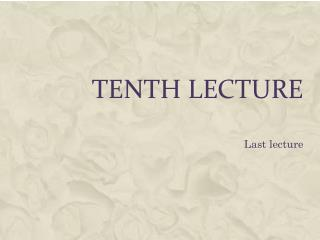DownloadDownload PresentationTenth lecture

# Tenth lecture

Télécharger la présentation## Tenth lecture

- - - - - - - - - - - - - - - - - - - - - - - - - - - E N D - - - - - - - - - - - - - - - - - - - - - - - - - - -
##### Presentation Transcript

1. Tenth lecture Last lecture

3. Shortly after the discovery of x-rays in 1885 by Professor Roentgen scientists began to notice the harmful effects of exposure to this radiation. There were many years before people realized how dangerous x-rays and other radiation could be. Quite number of pioneer radiologists suffered severe injuries, and some even died, as a result of prolonged exposure to dangerously high intensities of X-rays. These early workers in the field had no means of measuring the harm caused by radiation accurately and depended on unreliable effects such as the degree of skin reddening caused by the exposure, or on timing the exposure from a certain type of X-ray machine to establish quantity. • In order to evaluate the hazards of radiation it is necessary to have a measure of the harm which is the radiation cause. The radiation effect is measured in terms of exposure or dose

4. A) Exposure • One of the earliest observed properties of X-rays was their ability to ionize air. In 1928 the International Congress of Radiology specified this property as means of measuring the amount of X-radiation, and defined a unit which was named the roentgen, (R). • 1 R = exposure to X-rays or gamma-rays of such intensity that the electrons produced by this radiation in 1cm3 of dry air, at standard temperature and pressure, generate along their tracks electron - ion pairs carrying a total charge of 1esu of either sign

5. The SI unit of exposure is defined as 1 C / Kg air, without any new name proposed for it. Numerically • 1R = 2.58 x 10-4 C/Kg air • The roentgen suffers from two limitations, first it was defined in terms of electromagnetic radiation only, and secondly it was defined in terms of air only.

6. B) Absorbed Dose • Because of the limitation of roentgen (R), another unit called the radiation absorbed does or rad was defined as energy absorbed per unit mass of material. So, Where D is the absorbed dose and is the mean energy imparted by ionizing radiation to matter in a volume element and dm is the mass of matter in the volume element. The unit of the absorbed dose is rad defined as, • 1 rad = 100 erg/g • The SI unit of absorbed dose is the Gray (Gy), defined as • 1 Gy = 1 J/Kg =100 rad

7. C) The Dose Equivalent • The units of absorbed dose defined in the pervious section are quite adequate for the quantitative assessment of the effects of radiation to inanimate objects, like irradiated transistors or reactor fuel. For protection of people, however, the important thing is not the measurement of energy deposited (the absorbed dose), but the biological effects due to radiation exposure. Unfortunately, biological effects and absorbed dose do not always have one-to-one correspondence, and for this reason a new unit had to be defined, a unit that takes into accounts the biological effects of radiation.

8. The first step toward that task was the introduction of a factor called the relative biological effectiveness (RBE), defined as

9. In the meaning of RBE, there are the following notes: • RBE is defined in terms of photons; therefore, it follows that RBE=1 for electromagnetic radiation. Also, although the definition of RBE specifies the energy of the photons to be 200–300 KeV, RBE is taken as equal to 1 for photons of all energy. • A given type of radiation does not have a single RBE, because RBE values depend on the energy of radiation, the cell, the biological effect being studied, the total does, dose rate and other factors. • It is a well-known that the biological damage increase as the energy deposited per unit distance, the linear energy transfer (LET), increases. Thus, heavier particles (alphas, heavy ions, fission fragments) are, for the same absorbed dose, more biologically damaging than photons, electrons and positrons.

10. In 1963, the International Commission on Radiological Units and Measurements (ICRU) proposed the replacement of RBE by a new factor named the quality factor (QF). In 1973 the ICRU (82) recommended dropping "F" from QF, a suggestion that has now become practice. In 1977 the International Commission on Radiological Protection (ICRP) recommended that the dose equivalent (H) at a point in tissue be written as: H = NQD Where Q = Quality factor its values are given in Table 1.1. D = absorbed dose N= product of all the modifying factors. The suggested value of N is 1 When the unit of absorbed dose is multiplied by the corresponding Q value, the unit of dose equivalent (H) is obtained. The H units are 1 rem = Q x 1 rad and the SI unit, 1 Sievert (Sv) = Q x 1 Gy Thus, 1 Sv = 100 rem.

11. Table 1.1 Quality Factors for Several Types of Radiation